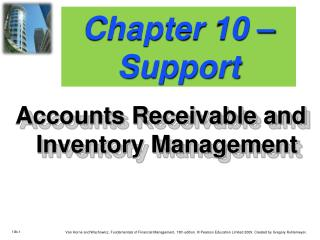DownloadDownload PresentationChapter 10 – Support

# Chapter 10 – Support

Télécharger la présentation## Chapter 10 – Support

- - - - - - - - - - - - - - - - - - - - - - - - - - - E N D - - - - - - - - - - - - - - - - - - - - - - - - - - -
##### Presentation Transcript

1. Chapter 10 – Support Accounts Receivable and Inventory Management

2. Remember? Credit and Collection Policies of the Firm Quality of Trade Account Length of Credit Period (1) Average Collection Period (2) Bad-debt Losses Firm Collection Program Possible Cash Discount

3. Example of Relaxing Credit Standards • The firm is currently producing a single product with variable costs of \$20 and selling price of \$25. • Relaxing credit standards is not expected to affect current customer payment habits. • Additional annual credit sales of \$120,000 and an average collection period for new accounts of 3 months is expected. • The before-tax opportunity cost for each dollar of funds “tied-up” in additional receivables is 20%.

4. Example of Relaxing Credit Standards Review how we can use Excel to analyze this type of problem (see ‘Relax Standards’ tab)! The \$24,000 gain exceeded the \$4,800 cost for a net benefit of \$19,200 so make the change! Now make some changes using Excel. Will you make the same decision if an increase of only 3,000 units occurs and it takes 4 months to collect?

5. Remember? Credit and Collection Policies of the Firm Quality of Trade Account Length of Credit Period (1) Average Collection Period (2) Bad-debt Losses Firm Collection Program Possible Cash Discount

6. Example of Relaxing the Credit Period Basket Wonders is considering changing its credit period from “net 30” to “net 60”. • The firm is currently producing a single product with variable costs of \$20 and a selling price of \$25. • Additional annual credit sales of \$250,000 from new customers are forecasted, in addition to the current \$2 million in annual credit sales. • The before-tax opportunity cost for each dollar of funds “tied-up” in additional receivables is 20%.

7. Example of Relaxing Credit Standards Review how we can use Excel to analyze this type of problem (See ‘Longer Credit Pd’ tab)! The \$50,000 gain exceeded the \$40,00 cost for a net benefit of \$10,000 so make the change! Now make some changes using Excel. Will you make the same decision if an increase of 12,000 units occurs because the firm allows customers to take 75 days to pay (4.8 turns)?

8. Remember?How Much to Order? • The optimal quantity to order depends on: Forecast usage Ordering cost Carrying cost Let us use Excel to analyze! Refer to ‘EOQ’ tab.

9. Total Inventory Costs • Total inventory costs (T) = • C (Q / 2) + O (S / Q) • O(S/Q): This is the ordering cost portion • C(Q/2): This is the carry cost portion • T = O(S/Q) + C(Q/2) or the total cost • Let us use Excel to look at total costs depending on our order size (See ‘EOQ’ tab)

10. Remember? Example of the Economic Order Quantity • 10,000 yards of fabric were used at a constant rate last period. • Each order represents an ordering cost of \$200. • Carrying costs are \$1 per yard over the 100-day planning period. What is the total costs per period if the quantity ordered varies from 200 to 6,000 units per order?

11. Economic Order Quantity When we solved the economic order quantity we found 2,000 units to be optimal – just like our formula told us. Observations: We find the optimal minimization that is identical We see that if we are off a small amount, that it is not making a major difference in total costs Try these changes and review the impact: Increase the carrying costs to \$2 per unit – expect more orders if holding inventory costs more, which would be a lower Q*. Increase the order costs to \$400 per order – expect fewer orders if it is expensive to place orders, which is a higher Q*. (Return carrying costs back to \$1)